## Pages

### MS1. Dirac delta functions

In mathematics, the Dirac delta function, or δ function, is a generalized function, or distribution, on the real number line that is zero everywhere except at zero, with an integral of one over the entire real line.The delta function is sometimes thought of as an infinitely high, infinitely thin spike at the origin, with total area one under the spike, and physically represents the density of an idealized point mass or point charge. It was introduced by theoretical physicist Paul Dirac. In the context of signal processing it is often referred to as the unit impulse symbol (or function). Its discrete analog is the Kronecker delta function, which is usually defined on a discrete domain and takes values 0 and 1.
(source: wikipedia )

The Dirac delta function is defined by the properties:

with,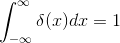We can say that the second property comes from the fact that delta function $\delta (x)$ represents the derivatives of the Heaviside Function $H(x)$.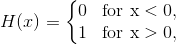Here's the pictorial representations of Heaviside and Dirac delta functions,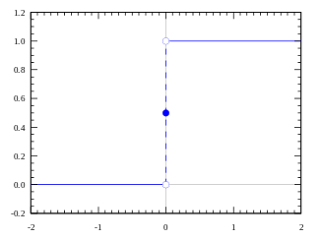Heaviside Unit step function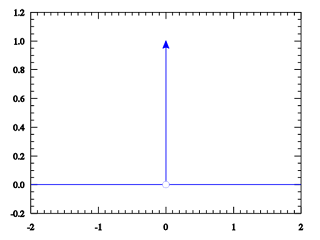Dirac Delta function
Keeping these two figures as above clearly shows that infact the dirac delta function is indeed the derivative of the heaviside unit step functions. That is the derivative of the heaviside functions is zero for $x\neq 0$. At $x=0$ the derivative is undefined.

We now look at some properties of the dirac delta functions,

1.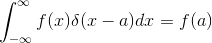2.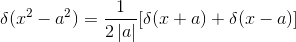3.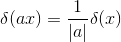4.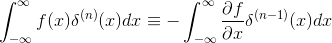5.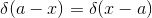For references on the dirac delta function and its properties you can read these,

wolfram notes
wikipedia notes